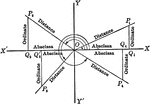### Reference Angles/Triangles Formed by Angles in Quadrants With Labels

Lines drawn to horizontal to form triangle ratios (reference triangles, angles). Axes, quadrants, abscissa,…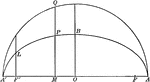### Ordinate and Major Axis of Ellipse

Illustration of half of an ellipse. The square of the ordinate of a point in an ellipse is to the product…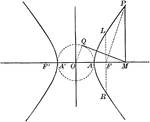### Auxiliary Circle and Hyperbola

Illustration of a hyperbola and its auxiliary circle. "Any ordinate of a hyperbola is to the tangent…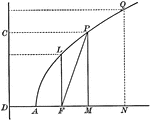### Parabola With Coordinates and Latus Rectum

Illustration of a parabola showing that any point of a parabola is the mean proportional between the…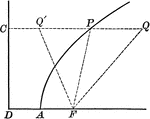### Point on Parabola

Illustration of a parabola showing point P is equidistant to the focus and the directrix.### Point on Parabola

Illustration of a parabola. "If a line PT is drawn from any point P of the curve, bisecting the angle…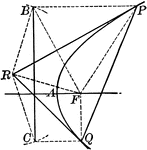### Tangent to a Parabola

Illustration of a tangent line drawn from an external point to a parabola.